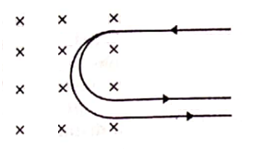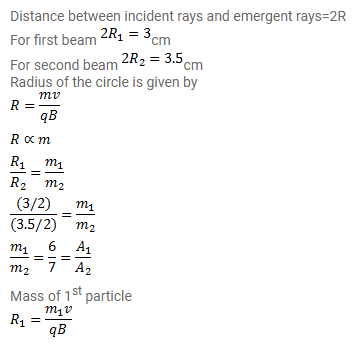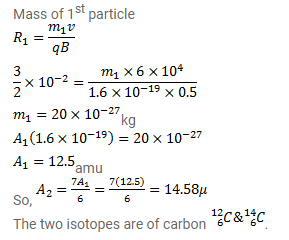# A narrow-beam of singly-charged carbon ions,

Question:

A narrow-beam of singly-charged carbon ions, moving at a constant velocity of $6.0 \times 10^{4} \mathrm{~m} / \mathrm{s}$, is sent perpendicularly in a rectangular region having uniform magnetic field $B=0.5 T$. It is found that two beams emerge from the field in the backward direction, the separations from the incident beam being $3.0 \mathrm{~cm}$ and $3.5 \mathrm{~cm}$. Identify the isotopes present in the ion beam. Take the mass of an ion $=A\left(1.6 \times 10^{-27}\right)_{\mathrm{kg}}$, where $\mathrm{A}$ is the mass number.Solution: